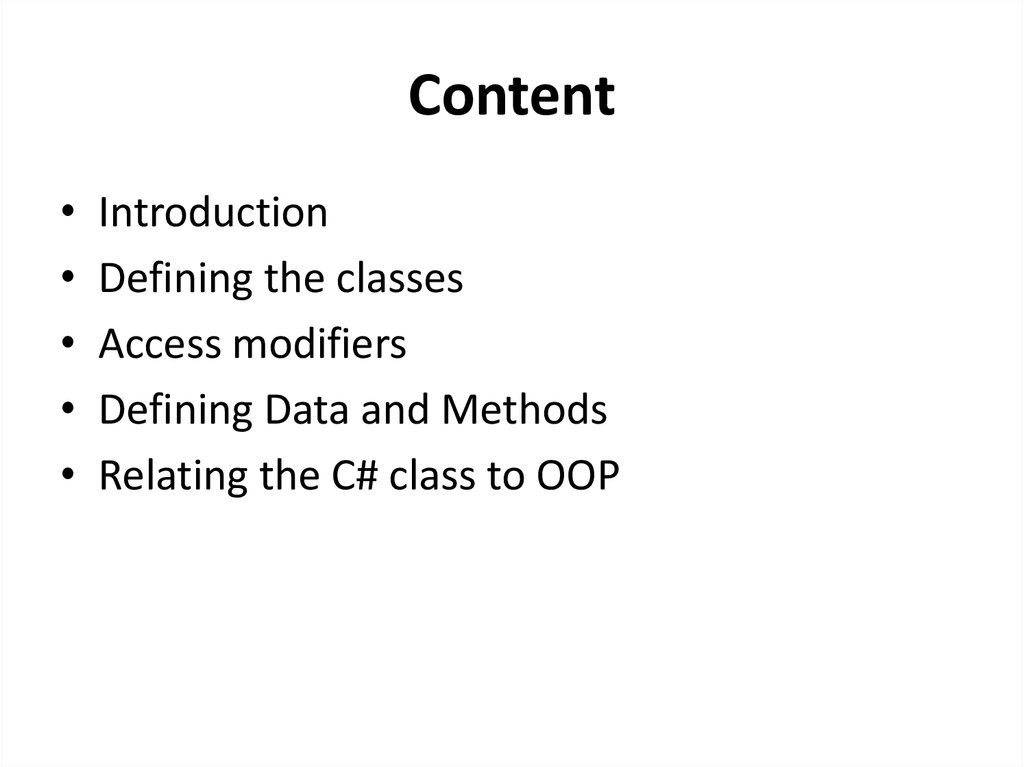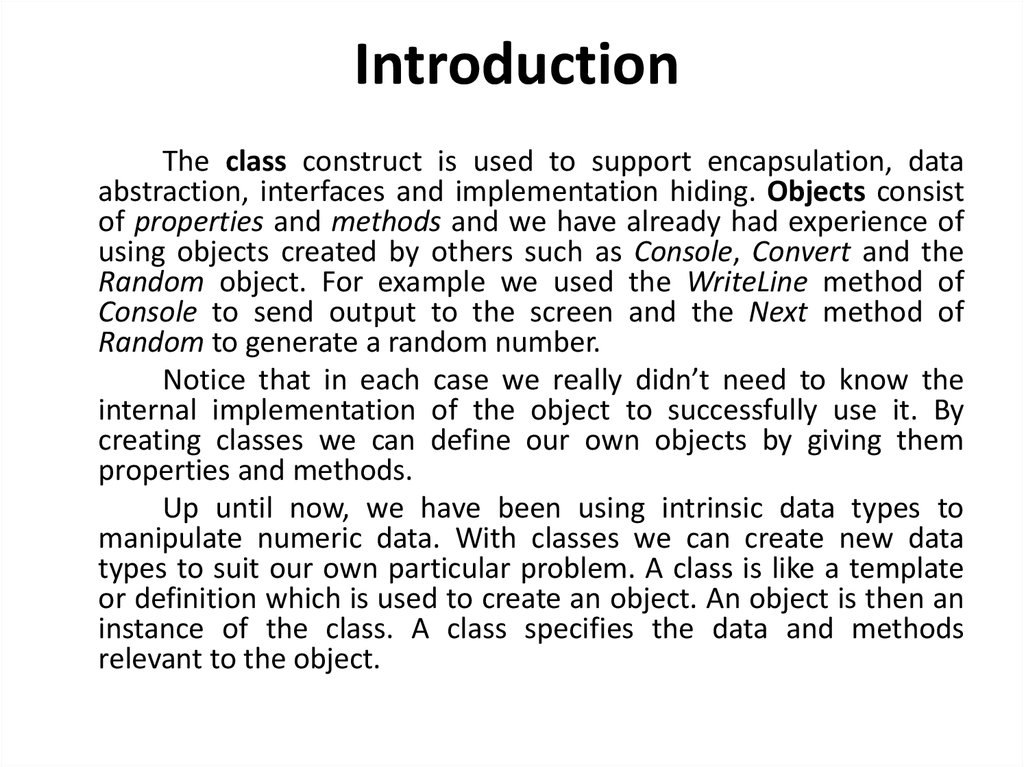# Introducing the C# Class Types. Defining classes, objects and methods

## 1. Object-oriented Programming

Lecture 4
Introducing the C# Class Types.
Defining classes, objects and methods.

## 2. Content

Introduction
Defining the classes
Access modifiers
Defining Data and Methods
Relating the C# class to OOP

## 3. Introduction

The class construct is used to support encapsulation, data
abstraction, interfaces and implementation hiding. Objects consist
using objects created by others such as Console, Convert and the
Random object. For example we used the WriteLine method of
Console to send output to the screen and the Next method of
Random to generate a random number.
Notice that in each case we really didn’t need to know the
internal implementation of the object to successfully use it. By
creating classes we can define our own objects by giving them
properties and methods.
Up until now, we have been using intrinsic data types to
manipulate numeric data. With classes we can create new data
types to suit our own particular problem. A class is like a template
or definition which is used to create an object. An object is then an
instance of the class. A class specifies the data and methods
relevant to the object.

## 4. Defining the classes

C# classes enable programmers to model real-world objects as objects in a
program which have properties and methods.
A class creates a new data type in C#.
The keyword class is used in C# to declare a class.
syntax:
[access-modifier] class identifier [:base-class]
{
class-body
}
Items in square brackets are optional.
Access modifiers are discussed later and typically the public keyword is used with
classes.
Identifier gives the class a name.
The optional base class is for advanced classes dealt with later on.
Finally the class body is where the data and methods are placed.

## 5. Access Modifiers

In general terms a class consists of a hidden part and an exposed
part, which users of the object can "see" or use. In the hidden part there
is private data and private methods, and the user of an object cannot see
or use these data or methods, this is equivalent to hiding the
implementation details. Public methods have their internals hidden from
the user, however, the user can invoke these methods. Public properties
expose the private data and provide a controlled interface whereby users
can modify the private data.
Access modifiers are placed before data and methods in the class
body. The two most commonly used are public and private. Public means
that users of the object either have direct access to data or can invoke a
method.
Private means that object users cannot directly access data or invoke the
method, essentially hiding them.

## 6. Defining Data

Data is declared inside the class body in almost exactly
the same way as we have seen already.
syntax:
[access-modifier] data-type identifier [= intial-value];
For example
private int x = 10;
The variable x is hidden, whereas the variable radius is
usable.

## 7. Defining Methods

Methods define the behavior of an object and are where we encapsulate pieces
of useful functionality that can operate on class data (both public and private). In
addition, data may be passed into methods as optional arguments and data may
also be returned by the method (i.e. the result of the manipulation).
syntax:
[access-modifier] return-data-type identifier ([data-type param1], [data-type
param2],...)
{
method-body
};
Example 1:
public int add(int a, int b)
{
int result;
result = a + b;
return result;
}
Example 2:
public double getP()
{
return 3.14;
}

## 8. Defining Methods

If no data is to be returned from a method call then the
keyword void is used.
Examples:
private void zero()
{
real = 0.0;
imag = 0.0;
}
public void set_real(double r)
{
real = r;
}

## 9. Putting it All Together

The following example is a first attempt at
implementing a complex class. It illustrates the
fundamental syntax of the class construct, with
private data, a private method and three public
methods.

## 10.

public class Area{
//data section
private double real = 0;
private double imag = 0;
//method section
private void zero()
{
real = 0.0;
imag = 0.0;
}
public void set_real(double r)
{
real = r;
}
public void set_imag(double i)
{
imag = i;
}
public double magnitude()
{
return Math.Sqrt(real * real + imag *
imag);
}
}
namespace MyComplex
{
class TestClass
{
static void Main(string[] args)
{
double r;
complex c = new complex();
c.set_imag(2);
c.set_real(-2);
r = c.magnitude();
Console.WriteLine("the magnitude is : {0}", r);
}
}
class complex
{…}
}

## 11.

namespace ConsoleApplication3
{
class Program
{
static void Main(string[] args)
{
Area a = new Area();
double areaRect = a.rectangle(4, 3);
double areaCirc = a.circle(7);
Console.WriteLine("rectangle S={0}, circle S={1}", areaRect,areaCirc);
}
{
Random r = new Random();
return r.Next();
}
}
class Area
{
//data section
private double length, width;
private double p = 3.14;
//method section
private void zero()
{
length =width = 0;
}
public double rectangle(double x, double y)
{
line1 = x;
line2 = y;
return x * y;
}
public double circle(double R)
{
return p * (R * R);
}
}
}

## 12. A reference object

A complex object is created by:
complex c = new complex();
However we could also break it down to:
complex c;
c = new complex();
This is because the complex c part is creating a reference object
which will refer to a complex object (it itself is not the complex
object). The new complex() part creates a complex object in
memory and returns a reference to it.
In other words if wrote:
new complex();
a new unnamed complex object would be created in memory but I
would have no way of modifying it later because I have no
reference to it. Therefore the two operations go hand in hand.

## 13. Relating the C# class to OOP

Let’s see how C# classes support these object oriented notions.
A class is a template for creating objects and in C# the class construct
is exactly that.
Objects are entities with properties and methods; in C# a class
contains data members corresponding to properties and methods
corresponding to OO methods.
Encapsulation is the process of hiding the implementation details of
an object; in C# the private access modifier is used to create hidden
data and methods (i.e. these are not directly available to the user of
an object).